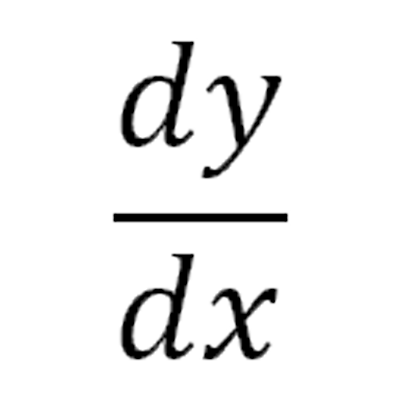Calculus: The Basics

A More In-Depth Guide to DerivativesThis symbol, dy over dx, represents a derivative.

Derivatives

From a mathematical standpoint, derivatives are based on slopes of functions at an exact point in time, independent from an average rate of change. Deribatives look at an exact point in time, focusing in on an instantaneous rate of change of some system. Derivatives can be used to describe rates of change in production, consumption, and of course position, velocity, and acceleration.

The derivative is a very versatile aspect of calculus.

Derivatives, and Calculus in general, were invented by two separte people individually. One person was Isaac Newton, and the other was Gottfried Wilhelm Leibniz. Both worked separate from one another and came up with nearly identical notions of calculus, especially the derivative.

What Can Derivatives be Used to Find?

• Slopes
• Extrema
• Maxima
• Minima
• Change
• Zeroes